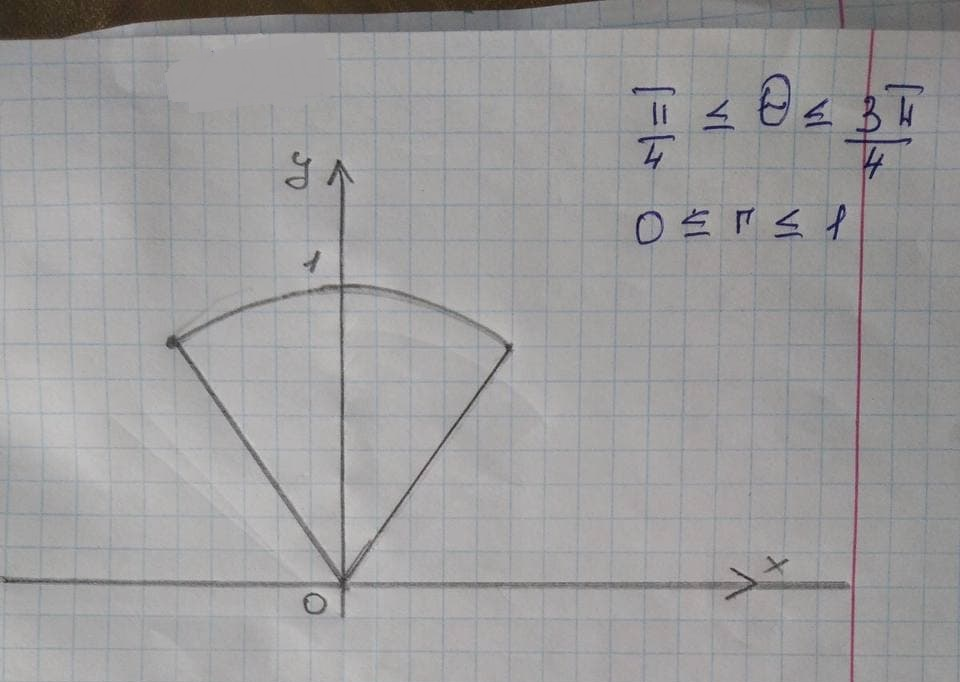# Graph the sets of points whose polar coordinates satisfy thevousetmoiec 2021-11-23 Answered
Graph the sets of points whose polar coordinates satisfy the given polar equation.
The given polar equation is written as follows:
$$\displaystyle{\frac{{\pi}}{{{4}}}}\le\theta\le{\frac{{{3}\pi}}{{{4}}}},{0}\le{r}\le{1}$$

• Questions are typically answered in as fast as 30 minutes

### Plainmath recommends

• Get a detailed answer even on the hardest topics.
• Ask an expert for a step-by-step guidance to learn to do it yourself.William Yazzie

Consider the polar coordinates as P(r, 0).
Here, r is the directed distance from origin O to point Pand $$\theta$$ is the directed angle from initial ray to OP.
From the given equation, the value of $$\theta$$ varies from $$\displaystyle{\frac{{\pi}}{{{4}}}}\to{\frac{{{3}\pi}}{{{4}}}}$$ and r varies from 0 to 1.
Polar point traces a circle when r is fixed at constant value and it traces a straight line when $$\theta$$ is fixed at constant value.
From the analysis, draw the sets of points whose polar coordinates satisfy the given polar equation as shown in Figure 1.